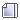###Author Topic: SPSS admission(3rd batch)  (Read 2898 times)

####zaidi« Reply #15 on: October 10, 2010, 12:46:48 PM »
My dear students  thank you very much for your various queries about SPSS course. The goals and learning outcomes  are given below:

course goals:
This course provide students with:
01. The ability to use common statistical technique to analyze experimental data so as to reach objective conclusions based on the obtained data.
02.  The ability to understand research reports.
03.  A basic introduction to using statistical software for data summerization and analysis.

Learning outcomes:

The students will be able to use basic mathmetical skills, incorporating them into various statistical procedures, and will be able to:
Define basic statistical terms.
Describe the four measurement scales.
Construct frequency distributions and represent them graphically.
Define and calculate percentile ranks and percentiles.
Define and calculate the measures of central tendency to include mode, median and mean.
Define and calculate the measures of variability to include the variance and standard deviation.
Explain the basic concepts of probability and discuss the importance of probability in statistics.
Explain the basic concepts of sampling theory to include sampling distributions.
Explain the basic concepts of hypothesis to include the null hypothesis, significance and errors in decisions concerning the null hypothesis.
Define correlation, calculate the appropriate correlation coefficients for interval and ordinal data, and use the obtained correlation coefficient to predict unknown values using linear regression.

NOTE: Dear students you are not in a position to understand all the things and take right decision....so you should talk to your course teacher's(ie...Marketing, Finance, Management and accounting) and i think they will help you in this regard.

A.K.M.Zaidi Satter
Lecturer, BBA Department
DIU

####ashiqbest012